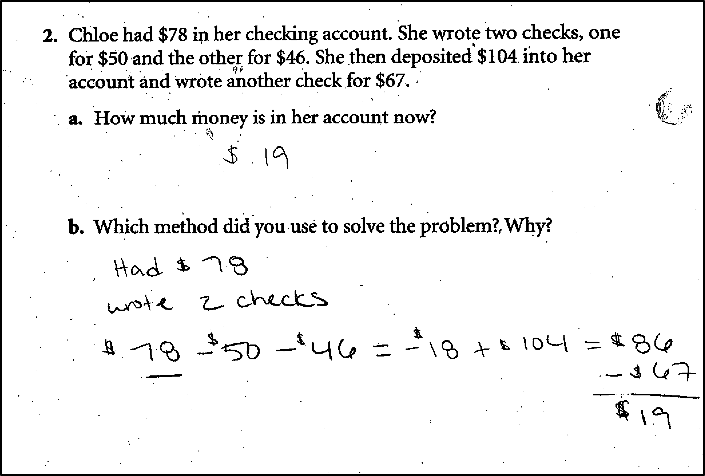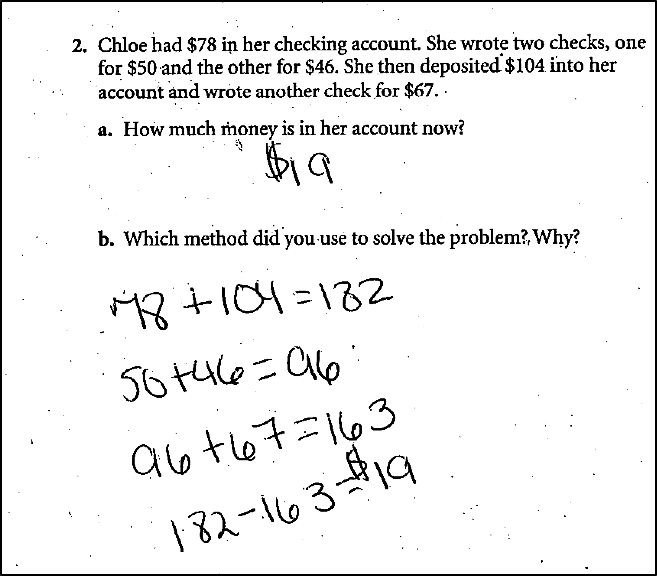## Paying Attention to Student Work

by Melissa Braaten

Effective teaching of mathematics uses evidence of student thinking to assess progress toward mathematical understanding and to adjust instruction continually in ways that support and extend learning.  National Council of Teachers of Mathematics, 2014

The quote above is a good description of “formative assessment” in mathematics, in which assessment information is sought for the purpose of informing and modifying instruction.  Paying attention to the work our students are handing in can provide some of this valuable “evidence” of student thinking.  But what does that really look like?  How much can you glean from a few things that a student writes on paper?

It can be helpful for teachers to have a structure to guide their analysis of student work. One of these methods is known as the DEN process, which stands for Describe, Evaluate, and Next Steps. The idea is to observe carefully and thoroughly (Describe), before making inferences about what the student may or may not understand (Evaluate). Lastly, the teacher plans her Next Steps with that student to untangle misunderstandings or push conceptual understanding further.

Let’s look at an example of how even a small sample of student work could provide insight and potential next steps for a teacher. The two samples below come from an adult education class at an intermediate level (GLE 5-8). Both students answered the question correctly, but looking closely at their work provides details about their understanding of operations, integers, and notation.

STUDENT ADescribe

In this sample, the student has recorded calculations horizontally, except for the last one. The last calculation is recorded vertically, but not lined up. The student has used subtraction to record the checks and addition to record the deposit, and has also included an equal sign after each step in the calculations. All the expressions are connected by these equal signs.

Evaluate

The student uses the equal sign the way it would be used on a calculator, to indicate the “answer” or result of a calculation, rather than to indicate equality between expressions (since the connected expressions are not equal). The final calculation was most likely written that way because the student was running out of room, not because she used the vertical format to perform the calculation, seeing as the place values are not aligned and there is no evidence of borrowing or other strategies which would be necessary for that particular subtraction problem. Although the student used a negative integer (-18) to represent when the balance of the account was in debt, she was more comfortable recording the check transactions using subtraction, rather than as negative integers. The student followed the chronology of the problem very carefully, even to the point of including the two checks together in the first calculation (probably because the existence of two checks is mentioned before the amounts).

Next Steps

This student does need some instruction in the proper use of the equal sign, since an understanding of equal as equality or balance between two expressions is critical for algebraic manipulations. In addition, this student could be encouraged to think about other ways that the problem could have been solved. While calculating each step chronologically works fine for this situation, there are many scenarios and word problems in which it is advantageous to work the numbers out of the order in which they are mentioned. Using negative integers instead of subtraction could help with this, since the addition of positive and negative integers can be done in any order.

STUDENT BDescribe

The student added the initial amount and the deposit, then (in two separate steps) added the three check amounts. Lastly, the student subtracted the check amount from the total of the initial amount plus deposit. Each calculation was recorded as a separate equation with a single number on the right.  The check amounts were represented as positive.

Evaluate

Student B appears to be more flexible than Student A in approaching the problem. Instead of calculating chronologically, she subtotaled the credits and the debits to the account. As a bonus, she never had to calculate a negative account balance or use negative integers at all. The notation is both horizontal and correct, but having the calculations broken up the way they are may indicate that the student is uncomfortable with writing multistep equations.

Next Steps

This student could work on combining the many steps of calculations into one multistep equation.  Teaching the student to use parentheses to group amounts in an equation would be helpful for representing the problem the way she solved it.

Although both students seemed to understand the word problem and answered it correctly, a closer examination still revealed areas in which a teacher might want to probe further.  Hopefully these examples illustrate the kind of rich formative assessment than can be gleaned from a very small sample of (correct) answers!

————————————————————————————————————————-

###### =============================================================Melissa Braaten is an adult education instructor at St. Mary’s Center for Women and Children in Dorchester, MA. Melissa has taught ASE and pre-ASE math and reading, as well as ABE writing, computer skills, and health classes. Melissa also is a training and curriculum development specialist for the SABES PD Center for Mathematics and Adult Numeracy at TERC.